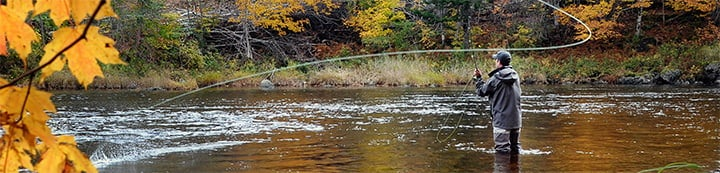1 - 5 of 5 Posts

#### CalSpey

·
##### Banned
Joined
·
28 Posts
Discussion Starter · ·
Is this a circle cast?
------------------------------------------------------------------------
-<<<<<<<<<<<<<<<<<
water flow
<<<<<<<<<<<<<
.(fly at dangle)
<me>
I left rod to 11, then 12 so rod is pointing straight up in air and continue to 3pm. Now line is upstream of me to my right.

<<<<<<<<<<<<<<<<<<<<<<
<<<<<<<<<<<<<<<<<<<<<<
<<<<<<<<<<<<<<<<<<<<<<< . (fly)
me

I then sweep the rod across my body, then sweep up to 11, so I have a d-loop. This works well with a rio grand on a snowbee 7/8 unless all the line is out, then I still have to practice swinging all this line into a d-loop

#### Moose

·
##### Here we go again!
Joined
·
620 Posts
What river are you practicing on? Perhaps we could meet up.

#### CalSpey

·
##### Banned
Joined
·
28 Posts
Discussion Starter · ·
Moose said:
What river are you practicing on? Perhaps we could meet up.
Any day this week that is not raining on the low flow of the feather will work.

#### crobarr

·
##### Registered
Joined
·
141 Posts
i love the ascii artwork. :smokin: